# Semi-simple endomorphism

(diff) ← Older revision | Latest revision (diff) | Newer revision → (diff)
semi-simple linear transformation, of a vector spaceover a fieldAn endomorphismofwith the following property: For any-invariant subspaceofthere exists an-invariant subspace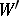such thatis the direct sum ofand. In other words,should be a semi-simple module over the ring,acting as. For example, any orthogonal, symmetric or skew-symmetric linear transformation of a finite-dimensional Euclidean space, and also any diagonalizable (i.e. representable by a diagonal matrix with respect to some basis) linear transformation of a finite-dimensional vector space, is a semi-simple endomorphism. The semi-simplicity of an endomorphism is preserved by passage to an invariant subspace, and to the quotient space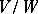.
Let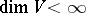. An endomorphism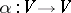is semi-simple if and only if its minimum polynomial (cf. Matrix) has no multiple factors. Letbe an extension of the fieldand letbe the extension of the endomorphismto the space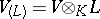. If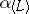is semi-simple, thenis also semi-simple, and ifis separable over, then the converse is true. An endomorphismis called absolutely semi-simple ifis semi-simple for any extension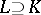; for this it is necessary and sufficient that the minimum polynomial has no multiple roots in the algebraic closure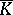of, that is, that the endomorphismis diagonalizable.